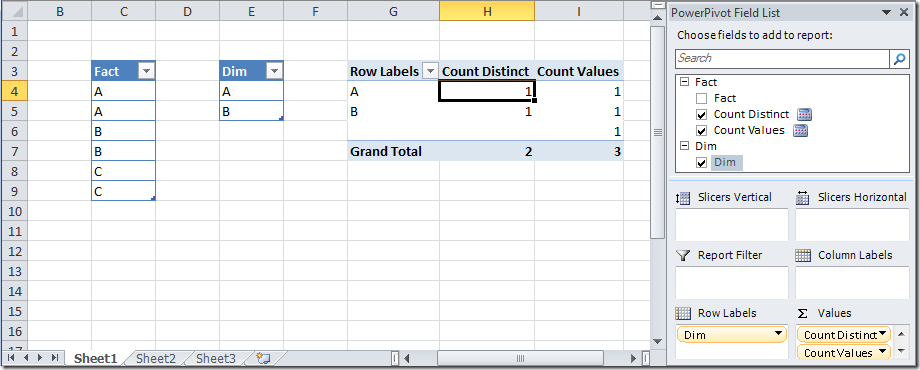I recently got a question about differences between DISTINCT and VALUES in DAX and thanks to Jeffrey Wang I created a simple example to describe the difference. Consider the two tables below: Fact and Dim tables, having a single column with the same name of the table. A relationship exists between Fact[Fact] and Dim[Dim]. This relationship generates a referential integrity violations in table Fact for rows containing C, which doesn’t exist in table Dim. In this case, an empty row is virtually inserted into the table Dim and all the rows in Fact that don’t have a correspondent member in Dim will point to this blank row. Such a row is the only difference between DISTINCT and VALUES.The measure “Count Distinct” is defined as =COUNTROWS(DISTINCT(Dim[Dim]))
The measure “Count Values” is defined as =COUNTROWS(VALUES(Dim[Dim]))

As you can see, the VALUES call returns the blank row in Dim that has been added to match unrelated rows in Fact, whereas the DISTINCT call only returns values that exists in the original Dim table. This is the only case when there is a difference in the behavior of these two functions. It is important to understand that a BLANK value existing in the original table, either in a source column or in a calculated column, will be considered by both VALUES and DISTINCT in the same way, as a regular member that will not be ignored by the DISTINCT function. You can see the extra blank value in the rows of the PivotTable in the screenshot above.

Not all the BLANK are the same in PowerPivot!

IMPORTANT UPDATE 04/14/2011

As you can read in the comments, if you want to calculate the number of non blank distinct values in a column, regardless of related values, a smart formula is:

= COUNTAX( DISTINCT( Dim[Dim] ), Dim[Dim] )

DISTINCTReturns a one column table that contains the distinct (unique) values in a column, for a column argument. Or multiple columns with distinct (unique) combination of values, for a table expression argument.

`DISTINCT ( <ColumnNameOrTableExpr> )`

VALUESWhen a column name is given, returns a single-column table of unique values. When a table name is given, returns a table with the same columns and all the rows of the table (including duplicates) with the additional blank row caused by an invalid relationship if present.

`VALUES ( <TableNameOrColumnName> )`

COUNTROWSCounts the number of rows in a table.

`COUNTROWS ( [<Table>] )`

BLANKReturns a blank.

`BLANK ( )`

COUNTAXCounts the number of values which result from evaluating an expression for each row of a table.

`COUNTAX ( <Table>, <Expression> )`